# Block designs for sequential block factors

## Introduction

Comparative experiments in agriculture and biology usually involve the estimation of treatment effects against a background of high non-treatment variability. Effective control of background variability is essential for good treatment estimation and the most common method of control is the block design. The aim of good block design is to group experimental units into homogeneous blocks where the precision of comparison within blocks is expected to be greater than the precision of comparison between blocks. Good block design should maximize the treatment information estimated within blocks and should minimize the treatment information confounded between blocks.

The most basic type of block design is the complete randomized blocks design where every treatment occurs in every block in proportion to its replication. The treatment replication need not be equal for all treatments but all treatments in the same block need to occur in some fixed proportion relative to their replication. Complete randomized blocks provide full efficiency on all treatment comparisons and are the designs of choice for experiments with a small number of treatments. However, because every block must contain every treatment, complete randomized blocks may be too large to give good within-block homogeneity of variance. In that situation, sub-division into smaller, more homogeneous incomplete blocks may be necessary to provide improved precision of estimation, especially for large field crop trials with many treatments.

Sometimes it can be assumed that two or more mutually orthogonal sets of crossed blocks such as the rows and the columns of a field trial will give improved control of nuisance effects. Often it is assumed that crossed block factors are mutually independent and that the block design can be chosen to minimize treatment information confounded both between the row blocks and between the column blocks independently. However, this is a very strong assumption and may well be unrealistic especially in large field trials where patterns of variability may not fit a simple additive row-and-column model.

Prior to the development of modern statistical software, the statistical analysis of experiments was a major constraint on the complexity of a design. Nowadays, modern software such as the R packages ‘lme4’ (Bates et al 2015) and lmerTest’ (Kuznetsova et al 2017) will allow efficient analysis of virtually any experimental design no matter how complex. Block designs have been extensively researched but often the emphasis has been to fit a relatively simple design for a pre-conceived blocks model. The modern paradigm for empirical model building is to add model terms until no further improvement in fit is possible judged against a suitable measure of model fit such as the Akaike information criterion (AIC) or the Bayesian information criterion (BIC). Empirical model building is commonly applied to treatment effects but there is no reason why the same approach should not be used for block effects. The aim of the ‘blocksdesign’ package is to provide a design of sufficient generality to ensure that the unknown ‘true’ block structure of an experiment to be effectively modelled at the analysis stage by empirical model fitting methods.

## Block sizes

A single set of complete replicate blocks will almost always improve the efficiency of an experiment but, for medium or large size experiments, further sub-division into smaller nested sub-blocks is often beneficial and resolvable incomplete block designs with a single level of nesting are widely used in agricultural research. An important study on the effects of block size on precision of estimation of treatments effects in large field trials was undertaken by Patterson and Hunter (1983). They reviewed 244 cereal variety trials arranged in generalized incomplete block designs and found that the mean improvement in yield efficiency due to the incomplete blocks was 1.43 relative to complete randomized blocks. Results varied greatly from one trial to another, however, and the distribution of efficiencies was positively skewed across the set of trials. Precision was more than doubled in one tenth of the trials and in another tenth the lattice arrangement had little effect on accuracy. Nevertheless, in half the trials the efficiency was 1.23 or more. They also modelled the background field variability of 166 of the trials assuming an empirical exponential variance function. Letting 2*phi(x) represent the estimated variance of the difference between two plots separated by a distance x, they calculated the semi-variance $$phi(x)$$ that best fitted the observed variances of the 166 trials to be:

$phi(x) = 0.209 (1 - 0.7250*942^{x})$

They then considered example designs for 48 varieties in three replicate blocks and estimated the theoretical variances of incomplete blocks of sizes 4, 6 or 8 based on the assumed semi-variance phi(x). They used these estimates to estimate the overall efficiency of the different block sizes including inter-block information and found the estimated efficiencies of block sizes 4, 6 and 8 were 1.460, 1.523 and 1.500 respectively. They concluded that for trials with up to about 64 treatments, the nested block size should be approximately equal to the square root of the number of treatments.

## Multi-level nesting

The Patterson & Hunter (1983) study compared different block sizes assuming different block designs each with a single level of nesting and a single nested block size. However, with multi-level nesting it is feasible to have a range of different block sizes in the same design so there is no reason why a design should be restricted to a single size of nested block. In particular, with a nested multi-stratum block design it is possible to include blocks of both size 8 and size 4 in the same design thus spanning the range of block sizes considered by Patterson & Hunter (1983). Modern methods of analysis allow for routine analysis of designs with multi-level nesting and we will examine a single design with three levels of nesting and three block sizes in the same design.

Assume a design for 3 replicates of 48 treatments with three complete replicate blocks of size 48, six nested blocks of size eight in each complete replicate block, two nested blocks of size four in each block of size eight and two nested blocks of size two in each block of size four. The ‘blocksdesign’ package (Edmondson 2017) can be used to fit a block design of this size by first fitting a set of three complete replicate blocks ($$reps$$), then fitting six blocks of size eight ($$sub1$$) nested within each replicate block, then fitting two blocks of size four ($$sub2$$) nested within each block of size eight and then finally fitting two blocks of size two ($$sub3$$) nested within each block of size four. Note that for nested block designs, the separate nested blocks must each have a unique block level.

Efficiency factors for nested blocks model
reps 2 1
reps+sub1 17 0.8769
reps+sub1+sub2 35 0.7275
reps+sub1+sub2+sub3 71 0.4113

The efficiency factors of the design can be extracted by using the $$\blocks\_model$$ extractor and the D-efficiencies of the four block sizes from the top stratum downwards were estimated to be 1, 0.877, 0.7275 and 0.4113. By comparison, the D-efficiencies of the same four block sizes from separate designs with a single nested level (not shown) were estimated to be: 1, 0.877, 0.7281 and 0.4118. The efficiencies were very slightly reduced in the third and fourth levels of the multi-level nested design relative to a single-level nested design with comparable block size but the reductions were very small and of no practical consequence. In our experience, the effect of repeated nesting on the efficiency of nested block designs is generally negligible although we have not made any formal study of this effect. NB the $$\blocks\_model$$ extractor provides efficiency factors for both an ‘Additive_model’ and a ‘Multiplicative_model’ but the ‘Multiplicative_model’ efficiency factors are intended to provide additional information about designs with crossed block factors and for fully nested block designs there should be no difference between the ‘Additive_model’ and the ‘Multiplicative_model’ efficiency factors.

## Simulated multi-level nesting

The efficiency of the multi-stratum example design discussed above was examined numerically by using 1000 simulated data sets based on the Patterson and Hunter (1983) $$phi(x)$$ function. The following four mixed models were fitted to each simulated data set using the ‘lmer’ function of the ‘lme4’ package (Bates et al. 2014):

1. A complete randomized blocks design with 3 complete main blocks of size 48
2. As above but with an additional set of 6 blocks of size 8 nested in each main block
3. As above but with an additional set of 2 blocks of size 4 nested in each block of size 8
4. As above but with an additional set of 2 blocks of size 2 nested in each block of size 4

All four models were fitted by maximum likelihood and for each simulation the Chi-square difference in fit between models 1 and 2,between models 2 and 3 and between models 3 and 4 each based on a single degree of freedom was calculated. Maximum likelihood models with nested random effects and the same fixed effects can be compared by a Chi-square deviance test and Figs 1-3 show histograms of the Chi-square differences between models 1 and 2, between models 2 and 3 and between models 3 and 4, respectively, based on the same 1000 simulations. Each comparison differs by a single level of nesting therefore the Chi-square deviances for each comparison has a single degree of freedom. However, because the variance components are constrained to be positive, the model is non-standard (Stram & Lee 1994) and the probability levels of the deviances do not follow the usual Chi-square distribution with 1 d.f. Instead, Dr. Hans-Peter Piepho, (University of Hohenheim; personal communication) recommends that significance tests should be implemented by halving the p-value of the standard Chi-square test on 1 d.f. Hence, the critical 5% test statistic for the deviance will be the 10% Chi-square critical value which is 2.706 on 1 d.f with a similar derivation for other critical p-values.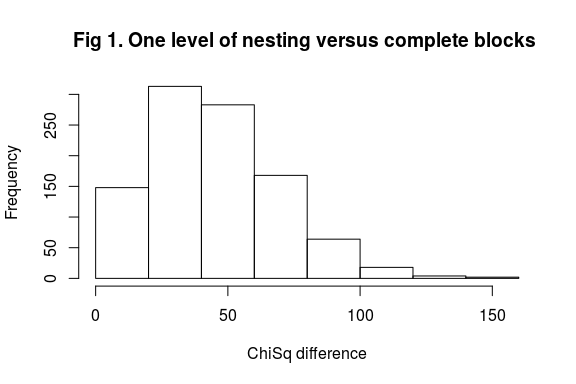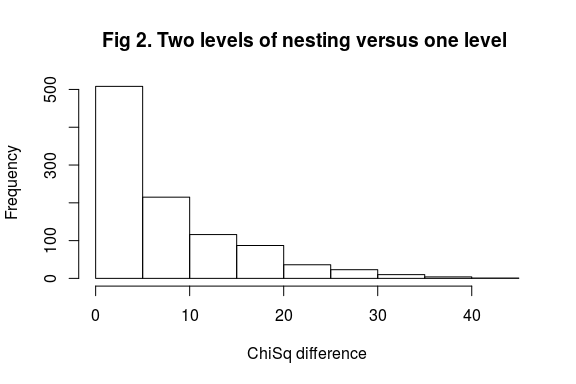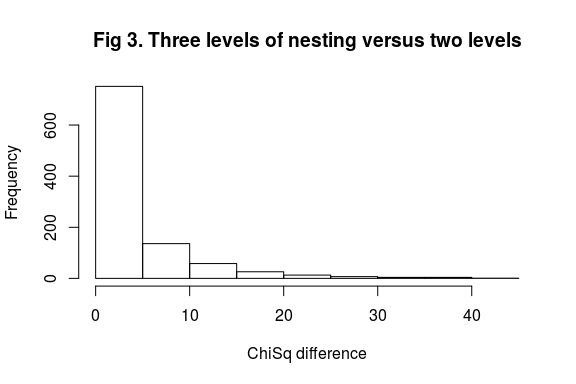Fig 1 shows that a single set of nested blocks of size eight gave a very significant improvement in model fit for the great majority of the simulated data sets (95% of simulations with Chi-square >10). Fig 2 shows that for almost half of the simulations, a second set of blocks of size four nested within each block of size eight gave a further large improvement in model fit (Chi-square>10) additional to the improvement in fit already achieved by the blocks of size eight. Finally, Fig. 3 shows that for approximately 25% of the simulations a third set of blocks of size two nested within each block of size four gave a significant and useful improvement in model fit additional to the improvement in fit already achieved by the first two sets of nested blocks. In summary, these results show that for large field experiments with spatial semi-variance function given by $$phi(x)$$ multi-level nesting can provide a substantial improvement in model fit of block effects compared with a single level of nesting.

## Crossed blocks designs

Where an experiment has two or more independent sources of background variability such as the rows and columns of a field crop trial, it can be desirable to accommodate both sources of variability simultaneously using a design with crossed block factors. The simplest crossed blocks design is a Latin square which is a $$v*v$$ row-and-column design for v replicates of v treatments. Each treatment occurs once in each row and once in each column and each row-by-column intersection contains a single plot. The rows and the columns provide two sets of mutually orthogonal blocks for the control of two separate independent sources of background variability and Latin squares have proved useful in a wide range of subject disciplines. However, Latin squares require as many replications as there are treatments and they quickly become impracticable as the number of treatments increases.

Latin squares can be generalized by relaxing the constraint that each row and each column must contain a complete set of treatments or by relaxing the constraint that each row and each column must intersect in a single plot. The first generalization allows classical row-and-column type designs with incomplete row blocks or incomplete column blocks while the second allows designs with incomplete blocks of treatments nested in individual row-by-column intersection. Examples of the first type of generalization are incomplete Latin squares (discussed in Cochran and Cox 1957) while examples of the second type are semi-Latin squares (discussed by Preece & Freeman 1983). An example of a row-and-column design that includes both types of generalization simultaneously is the incomplete Trojan square (Edmondson 1998).

The analysis of a generalized Latin square normally requires a mixed model type analysis. A full analysis of a generalized crossed blocks design requires a factorial analysis of the crossed block effects with an additive main effect for each crossed blocks factor, a 2-factor interaction for each pair of crossed blocks factors and so on for all possible higher-order crossed block interactions. Crossed blocks with a single plot in each crossed block intersection confound crossed block interactions with treatment effects and for these designs only the crossed blocks main effects are estimable. However, for crossed blocks with multiple plots in each intersection, the crossed block interactions can be estimated separately from the treatment effects and for these designs the block factor interactions can be estimated and included in the fitted model in the usual way.

## Crossed blocks: a literature example

Durban et. al. (2003) examined data from an experiment with two replicates of 272 spring barley varieties arranged in an array of 34 beds (east-west) and 16 rows (north-south). The experiment was designed as a row-and-column design with 16 rows and 34 columns subject to the constraint that rows 1-8 contained one complete set of treatment replicates while rows 9-16 contained the other set. Thus the original analysis of the block design had two fixed replicate block effects with 16 row and 34 column effects.

The assumptions underlying the analysis of this type of row-and-column design are that row and column effects are additive and that the residual variances after allowing for treatment and additive block effects are homogeneous. Durban et. al. (2003) showed, however, that after fitting the additive effects of rows, columns and replicates to the barley variety trial data, the residual plot variances were far from homogeneous.

To improve the basic row-and-column analysis, Durban et. al. (2003) considered fitting a semi-parametric model for loess smoothing (Hastie 2017) of local trend effects within individual row blocks. An alternative approach can be based on a multi-level crossed block design and in the following discussion, we use ‘post-blocking’ to show how the variety trial data can be explained by fitting a generalized row-and-column block structure. We then show how ‘blocksdesign’ can be used to construct generalized crossed block design that can accommodate a wide range of possible crossed block effects.

## Crossed blocks: multi-level analysis

In the following analysis, we partition the 34 columns of the variety trial data into a hierarchy of nested column blocks to provide better positional control of trends within rows. A maximal partition fits a hierarchy of two, four, eight, 16 and 34 nested column blocks but for this study we consider a partition into just three sizes of column blocks, a set of four large column blocks, $$Col1$$, of widths 9,8,8,9, a set of eight medium column blocks, $$Col2$$, of widths 5,4,4,4,4,4,4,5 and a set of 34 column blocks, $$Col3$$ each of width one. We first add the replicate block effects and then the row effects nested within replicates and then add the column blocks in decreasing size order from largest to smallest. For each size of column block, we first add the main effects of the column blocks and then, for the column blocks with width greater than one, we add the row-by-column interaction effects for that set of column blocks.

Sequentially fitted block model terms
Block model terms
(1 | Reps) + (1 | Rows)
(1 | Reps) + (1 | Rows) + (1 | Col1)
(1 | Reps) + (1 | Rows) + (1 | Col1) + (1 | Rows:Col1)
(1 | Reps) + (1 | Rows) + (1 | Col1) + (1 | Rows:Col1) + (1 | Col2)
(1 | Reps) + (1 | Rows) + (1 | Col1) + (1 | Rows:Col1) + (1 | Col2) + (1 | Rows:Col2)
(1 | Reps) + (1 | Rows) + (1 | Col1) + (1 | Rows:Col1) + (1 | Col2) + (1 | Rows:Col2) + (1 | Col3)

The models are fitted by the lme4 package using maximum likelihood and the improvement in fit due to each added column effect is assessed by comparing the model fit of each model with its preceding model using the various goodness of fit tests supplied by an $$anova()$$ of the complete set of models taken in order of fitting.

Improvement in model fit due to each added block effect
Model Df AIC BIC logLik deviance Chisq Chi Df Pr(>Chisq)
0 275 584.32 1766.5 -17.161 34.322
1 276 476.77 1663.3 37.615 -75.23 109.5521 1 < 2.2e-16 ***
2 277 429.72 1620.5 62.138 -124.276 49.0459 1 2.500e-12 ***
3 278 366.4 1561.5 94.801 -189.601 65.3248 1 6.352e-16 ***
4 279 363.16 1562.6 97.42 -194.841 5.2397 1 0.02208 *
5 280 282.81 1486.5 138.593 -277.187 82.3458 1 < 2.2e-16 ***

The maximum likelihood comparisons for the successively fitted column blocks show the effects of each sequentially added column block together with the respective row-by-column block interaction effects. The column blocks are added in descending order of size and the base model, Model 0, is the model with replicate block effects and complete row block effects but no fitted column block effects.

1. Model 1 tests the effects of the large sized column blocks and shows a very large improvement in model fit compared with the base model.
2. Model 2 tests the effects of the interactions between the large sized column blocks and the row blocks and shows a very large improvement in model fit compared with Model 1.
3. Model 3 tests the effects of the medium sized column blocks and shows a very large improvement in model fit compared with Model 2.
4. Model 4 tests the effects of the interactions between the medium sized column blocks and the row blocks and shows a significant but very small improvement in model fit compared with Model 3.
5. Model 5 tests the effects of the smallest sized column blocks of width one and shows a very large improvement in model fit compared with Model 4.

Based on these results, the best fitting model for the observed data includes the additive effects of the three sizes of column blocks $$Col1$$, $$Col2$$ and $$Col3$$ plus the interaction between the $$Col1$$ blocks and the row blocks. The interaction between the $$Col1$$ blocks and the row blocks shows that the column blocks of sizes 9,8,8,9 are not simply additive and this means that the model requires separate block effects for each nested row block in the $$Col1$$ stratum. The $$Col2$$ and $$Col3$$ column blocks are nested within the $$Col1$$ column blocks and simple additive row-and-column blocks are adequate for the $$Col2$$ and $$Col3$$ blocks nested within the $$Col1$$ blocks. The overall fitted model relaxes the strong assumption that the row and column effects must be additive over the full 34 beds width of the trial. The large interaction effect between the $$Col1$$ blocks and the row blocks shows that at the scale of the $$Col1$$ blocks, the assumption of additive row and column effects is invalid.

The goodness of fit of the original row-and-column model versus the best fitting row-and-column model is shown in Figures 4 and 5, respectively, where the block and treatment adjusted REML residuals of the original row-and-column analysis and the best fitting multi-level analysis are plotted against column position for each of the 16 rows of the experiment. Visual inspection shows that the original row-and-column analysis (Fig 4) fails to provide a good model fit whereas the best fitting multi-level analysis (Fig 5) provides an excellent model fit.

## Loading required package: Matrix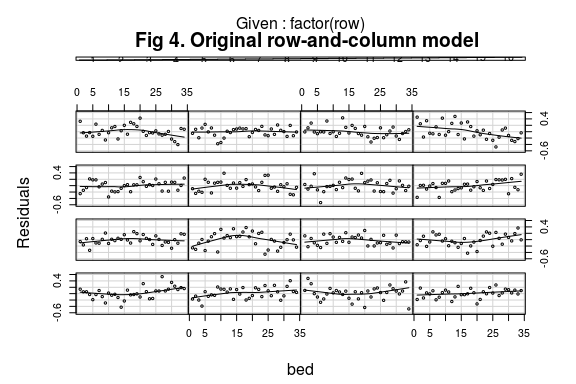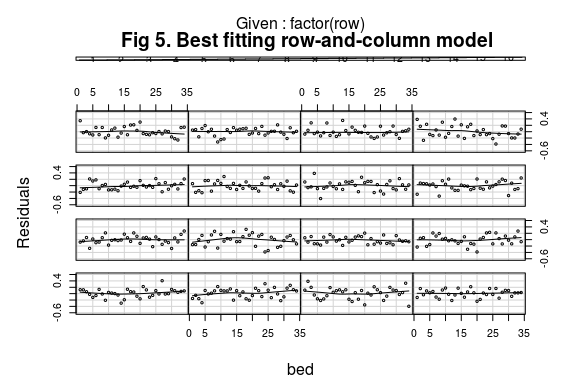## Construction of generalized crossed block designs

The post-blocked analysis of the spring barley variety trial shows that, for large field trials, a complex multi-level block analysis can substantially improve the precision of estimation of treatment effects. However, the original design of the trial assumed a simple conventional row-and column blocks design with 16 row blocks and 34 column blocks together with the constraint that one replicate was allocated to rows 1-8 and the second to rows 9-16. In practice, this means that the block structures used for the design are not fully efficient for the assumed post-blocked analysis.

As the assumption that rows and columns blocks are additive is not necessarily valid, it is usually desirable, where possible, to construct a design that is efficient both for the additive effects and for the interaction effects of the crossed block factors. However, except for certain special designs such as Trojan designs, it is not usually possible to optimize both the additive and the interaction effects of crossed block factors simultaneously.

Instead, the ‘blocksdesign’ package adopts a compromise approach by optimizing a weighted combination of an additive block effects information matrix and a multiplicative effects information matrix. If the weighting factor is set to zero, the design is optimized for additive effects only, whereas if the weighting factor is set to one, the design is optimized for the full multiplicative model. Intermediate weighting gives a compromise optimization that includes all the block factor effects in the optimization but with more importance attached to the additive effects when the weighting factor is small and more importance attached to the interaction effects when the weighting factor is large. The default weighting is 0.5.

The following ‘blocksdesign’ output shows the compromise post-blocked analysis of the barley variety trial with default weighting equal to 0.5. The design efficiency factors are extracted using the formula $$\blocks\_model$$ and for each design the efficiency factors show both the additive block effects and, where available, the multiplicative block interaction effects.

Efficiency factors for additive effects of crossed blocks model
Additive model DF weight=0 weight=.5 weight=1
Reps 1 1 1 1
Reps+Rows 15 0.9648 0.9648 0.9648
Reps+Rows+Col1 18 0.9605 0.9603 0.9573
Reps+Rows+Col1+Col2 22 0.9507 0.9506 0.9476
Reps+Rows+Col1+Col2+Col3 48 0.8875 0.8872 0.887
Efficiency factors for multiplicative effects of crossed blocks model
Multiplicative model DF weight=0 weight=.5 weight=1
Reps 1 1 1 1
Reps.Rows 15 0.9648 0.9648 0.9648
Reps.Rows.Col1 63 0.841 0.8441 0.8442
Reps.Rows.Col1.Col2 127 0.6749 0.6784 0.6785
Reps.Rows.Col1.Col2.Col3 543 NA NA NA

The efficiency factors for the compromise design (weighting = 0.5) should show additive efficiency factors close to those found for the fully additive design (weighting = 0) and multiplicative efficiency factors close to those found for the fully multiplicative design (weighting = 1). Comparison of the sets of efficiency factors for the three designs does, indeed, show that the compromise design is almost as efficient as the fully additive design for additive block effects and almost as efficient as the fully multiplicative design for multiplicative block effects. We can therefore conclude that a weighted design with a suitable weighting factor will provide a design that is robust for different assumptions about the fitted crossed blocks model at the analysis stage.

## Discussion

The advantages of simplicity, flexibility and robustness mean that block designs are the designs of choice for most agricultural experiments. Wherever possible, replicated experiments should be divided into complete replicate blocks and the complete replicate blocks should be used to account for large natural or non-treatment differences between the experimental units. For example, with large experiments cultural operations such as planting or harvesting that might occur over several days it is often useful to use the main blocks as management units so that all cultural operations on the same replicate block are carried out on the same day (Bailey 2008 Chapter 4). Sometimes the physical constraints of an experiment mean that the natural block size does not coincide with the size of a replicate block in which case the main blocks cannot be complete treatment replicates. In that situation, the best option is to allocate treatments to main blocks in such a way that the efficiency of the main block design is maximized (see Bailey 2008 Chapter 11).

The Patterson & Hunter (1983) paper examined the effects of different block sizes on the efficiency of resolvable nested block designs assuming an empirical exponential variance function for the correlations between plots within replicate blocks. They assumed a design with 3 replicates of 48 treatments and examined the efficiency of nested blocks of size 8 6 or 4, using different resolvable nested block design for each block size. In this vignette, the Patterson & Hunter (1983) example has been generalized by simulating the efficiencies of recursively nested blocks of size 8 and 4 in the same nested block design. The range of block sizes could be further extended by nesting an additional set of blocks to give blocks of size 2 in the bottom stratum of the design but that option will not be further explored here.

The trials assessed in the Patterson & Hunter (1983) paper were cereal trials mostly with long thin plots arranged side by side. This arrangement is convenient for one-dimensional blocking and most of the trials in the Patterson and Hunter study had one-dimensional blocks. Plots that are more nearly square are less easily blocked in one-dimension and may require two-dimensional blocks. Often, simple nested blocks are quite adequate for two-dimensional blocking provided that the blocks are as compact as possible in both dimensions.

The reason that the additive row-and-column analysis was so unsuccessful for the spring barley experiment in Durban et. al. (2003) is because, as shown in the maximum likelihood analysis of the various possible fitted models, there was a very large and significant interaction between the column blocks and the row blocks that was not properly accounted for in the original row-and-column analysis. After fitting a suitable interaction term between the column blocks and the row blocks, the resulting multi-level analysis gave an excellent fit to the observed data.

The outstanding problem in the design and analysis of complex multi-level block models is the issue of model selection. Muller et al. (2013) give an excellent review of model selection for linear mixed models at the analysis stage but the problem of model selection at the design stage involves different issues. Selection of a suitable blocks model at the design stage is critical for enabling the selection of a suitable blocks model at the analysis stage. The risks of selection bias and model over-fitting are well known and further work on the right balance between efficiency of estimation and the risk of over-fitting of nested block designs would be valuable.

Acknowledgement: I would like to thank Prof. Dr. Hans-Peter Piepho of the University of Hohenheim for much useful advice and encouragement to publish this paper.